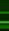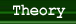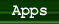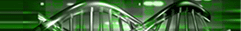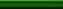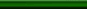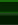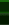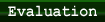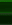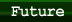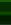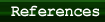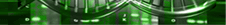### I. Solving NP-Complete problems

#### A. Definitions:

1)   A decision problem is in the class NP if there is no known algorithm for it that will execute in polynomial time on a conventional computer, and it can be solved in polynomial time using a non-deterministic computer (Miller).

2)   A problem is intractable if no polynomial time algorithm can possibly be devised for it (Miller). In other words, as the complexity of intractable problems increase, the time required to solve them increases at an exponential rate (Adams).

3)   A problem in NP is NP-complete if every other problem in NP can be expressed in terms of it by means of a polynomial time algorithm (Miller).

If any problem in NP is shown to be intractable then all NP-complete problems are intractable. However, if any NP-complete problem can be solved in polynomial time, all problems in NP become tractable (Miller).

#### B . How is it done?

A test tube of DNA strands can be used to simulate a non-deterministic computer using the techniques of molecular biology. Theoretically speaking, otherwise intractable problems can be implemented in polynomial time on this DNA computer. One example is Adleman’s simulation of the Traveling Salesman problem: known to be NP-complete, the problem had an execution time that was linear to the number of cities (Miller). However, Adleman’s method of the exhaustive search algorithm is able to solve problems in polynomial time only at the expense of exponential increases in volume (Adams).

#### C. Implications to real life problems

Particularly in business planning and management science, many of the cost optimization problems are NP-complete and currently solved using heuristic methods and other approximations. These problems include scheduling, routing, and optimal use of raw materials, and correspond to problems already solved, either theoretically or experimentally, using DNA computation (Adams).

### II. Cryptography

Currently, researchers have mapped a way in which DNA computers could be used to crack messages encoded with Data Encryption Standard (DES) (Bergquist). Although this problem has already been solved using conventional techniques in a much shorter time than proposed by the DNA methods, the DNA models are much more flexible, potent, and cost effective (Adams).

### III. Turing Machine

A number of theoretical models for creating a universal DNA computer have been developed, and mathematical proofs have shown that some models of DNA computing are at least equivalent to a classical Turing machine (Adams). In year 2001, Ehud Shapiro’s group at the Weizmann Institute in Israel built a programmable and autonomous computing machine using the DNA strands as inputs and the transition rules. Shapiro’s machine was a “special case of the Turing Machine: a two-state, two-symbol automaton” (Noble), but he is still hoping to develop a fully operational molecular Turing Machine in the future (Parker).

### IV. Patterning polysilicon directly without lithography

According to Adleman, by laying a grid of DNA precisely on top of silicon substrates, very dense patterns of nanocrystals can seed polysilicon circuits fabricated atop them. The accuracy of DNA enables circuits to be built on a chip, molecule by molecule, instead of with bulk deposition and lithography. Those substrates would pattern themselves onto flat sheets, which could then be used to direct the placement of polysilicon materials atop them, making up the actual circuit elements (Johnson).